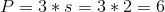## Example Questions

### Example Question #1 : Equilateral Triangles

What is the perimeter of an equilateral triangle with an area of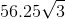?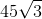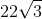Explanation:

Recall that from any vertex of an equilateral triangle, you can drop a height that is a bisector of that vertex as well as a bisector of the correlative side. This gives you the following figure: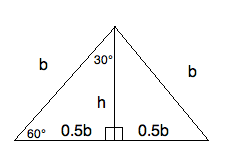Notice that the small triangles within the larger triangle are both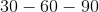triangles. Therefore, you can create a ratio to help you find.

The ratio of the small base to the height is the same as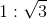.  Therefore, you can write the following equation: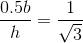This means that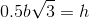.

Now, the area of a triangle can be written: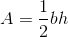, and based on our data, we can replacewith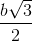.  This gives you: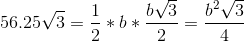Now, let's write that a bit more simply: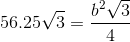Solve for. Begin by multiplying each side by: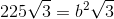Divide each side by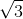: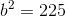Finally, take the square root of both sides. This gives you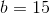. Therefore, the perimeter is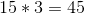.

### Example Question #1 : How To Find The Perimeter Of An Equilateral Triangle

An equilateral triangle with a perimeter of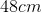has sides with what length?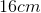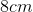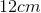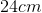Explanation:

An equilateral triangle has 3 equal length sides.

Therefore the perimeter equation is as follows,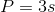.

So divide the perimeter by 3 to find the length of each side.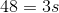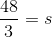### Example Question #141 : Act Math

Jill has an equilateral triangular garden with a base of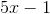and one leg with a length of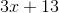, what is the perimeter?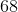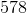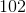Explanation:

Since the triangle is equilateral, the base and the legs are equal, so the first step is to set the two equations equal to each other. Start with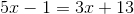, addto both sides giving you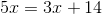. Subtractfrom both sides, leaving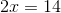. Finally divide both sides by, so you're left with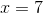. Plugback in forinto either of the equations so that you get a side length of. To find the perimeter, multiply the side length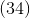, by, giving you.

### Example Question #1 : How To Find The Perimeter Of An Equilateral Triangle

Find the perimeter of an equilateral triangle whose side length is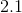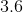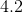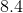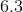Explanation:

To find perimeter of an quilateral triangle, simply multiply the side length by. Thus,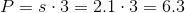### Example Question #2 : How To Find The Perimeter Of An Equilateral Triangle

Find the perimeter of an equilateral triangle whose side length is.Explanation:

To solve, simply multiply the side length by. Thus,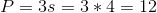### Example Question #1 : Equilateral Triangles

Find the perimeter of an equilateral triangle given side length of 2.